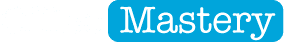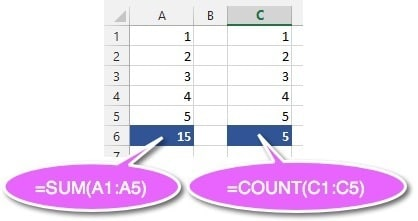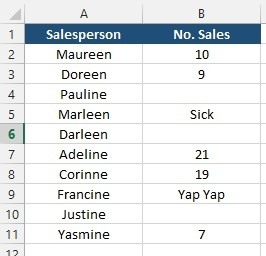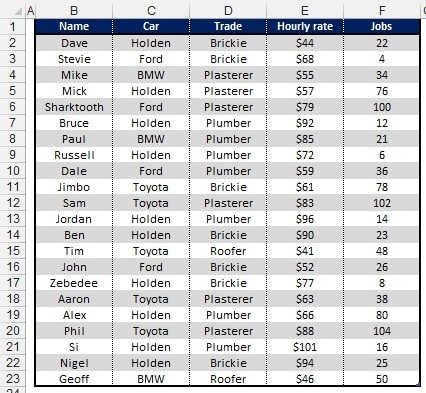COUNT vs SUM vs COUNTA vs COUNTBLANK vs COUNTIF# COUNT vs SUM vs COUNTA vs COUNTBLANK vs COUNTIFMany people get confused about how and when to use some of the basic Excel functions. For example, when do you use SUM and when do you use COUNT?

In a moment I’ll tackle that specific question.

Then I'll bring 3 other COUNT functions into the mix - COUNTA, COUNTBLANK and COUNTIF and show you where you would use each one.

Feature Download: Grab a printable PDF of everything in this post (completely free). Never forget a key point again!

## What is the difference between SUM and COUNT?

Very simply, SUM calculates a total for a number of cells or values, so it’s answering the question: HOW MUCH? Or, WHAT IS THE TOTAL?

COUNT tells you HOW MANY cells meet a certain condition.

Consider the following data:Figure 01: SUM vs COUNT

Cell A6 uses a SUM function to add up the values in cells A1 to A6.

Cell C6 uses a COUNT function to find how many cells in the range C1 to C6 contain numbers. The COUNT function ignores blank cells or cells that contain text or symbols.

## Other COUNT functions in Excel

There are number of other functions available in Excel. Heres a quick summary of what they do, followed by an example of each.

• COUNT counts how many cells in a range contain numeric data (numbers).
• COUNTA counts how many populated cells in a range (i.e. not blank).
• COUNTBLANK counts how many blank cells in a range.
• COUNTIF counts how many cells in a range meet a certain condition.

Consider the following data:Figure 02: Sales made by the sales team

Here’s the results for each formula:

###### =COUNTBLANK(B1:B11)

There is no single function that tells you the number of text cells but you can work it out with this formula:

###### =COUNTA(B1:B11) - COUNT(B1:B11)

To demonstrate the COUNTIF function, consider the following data:Figure 03: A table showing stats for some trades people

The COUNTIF function needs 2 bits of information - the range of cells you are looking at and what it is that you’re checking for. The criteria is always encapsulated in double quotation marks (“) and is not case sensitive.

## Example of the COUNTIF function

To find how many tradespeople drive a Toyota:

###### =COUNTIF(C2:C23,"Toyota")

To find how many plumbers there are:

###### =COUNTIF(D2:D23,"Plumber")

To find how many tradespeople charge more than \$70 per hour:

###### =COUNTIF(E2:E23,">70")

To find how many of the tradesmen’s names start in the last half of the alphabet:

###### =COUNTIF(B2:B23,">M")

I hope you found this post useful.

What do you think?